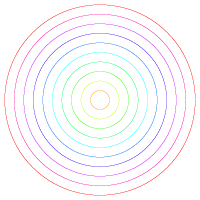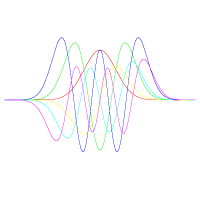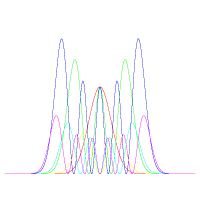Fall 2003

# Mathematics Math21b Fall 2003

## Linear Algebra and Differential Equations

Office: SciCtr 434
Email: knill@math.harvard.edu# The harmonic oscillator

## Classical Harmonic Oscillator```d2/dx2 f(x) = c2 f(x) ```
is an ordinary differential equation with solution
 ```f(x)= f(0) cos(c x) + (f'(0)/c) sin(c x). ```
It can be realized by masspoint attached to a spring. The constant c depends on the gravitational strength, as well as the spring constant. The differential equations can be rewritten as the linear system
 ```dx/dt = y dy/dt = - x ```
The flow leaves the level curves of the energy function H(x,y) = x2 + y2 invariant, which are circles.

## Quantum Harmonic OscillatorEigenfunctions fk and probability densities |fk|2. ``` f0[x_]:=Exp[-x^2/2]; A[g_]:=Function[y,-D[g[x],x]+x g[x] /. x->y]; l[f_]:=Integrate[f[x]^2,{x,-Infinity,Infinity}]; S[f_]:=Module[{},ff[x_]:=Evaluate[ Simplify[A[f][x]/l[f]]];ff]; f[0_]:=f0; f[k_]:=S[f[k-1]]; f[x] ```
The partial differential equation
 ```d/dt f(t) = i T(f) = i (-d2/dx2 f(x) + x2 f(x) ) ```
is called the Schrödinger equation for the quantum harmonic oscillator. For each eigenvalue Ln with eigenfunction fn of T, the time evolution is fn(t) = exp(i Ln t) fn(0). If f(0) = a1 f1 + a2 f2 + ... then
 ```f(t) = exp(i L1 t) f1(0) + exp(i L2 t) f2(0) + ... ```
We can write T(f) = P2 + Q2, where P(f)(x) = i f'(x) and Q(f) = x f(x)(x). The Hamiltonian T has the same structure as the Hamiltonian of the harmonic oscillator giving the quantum system the name "quantum harmonic oscillator".
T has the eigenvalues Ln = 1+2n, with eigenvectors fn, which are recursively be defined by fn+1 = (x-D) fn starting with f0(x)=exp(-x2/2). Simlilar as in Fourier theory, it is possible to write any function f for which |f|2 has a finite integral over the real line as a sum of such functions and so solve the Schrödinger evolution explicitely.
Back to the main page